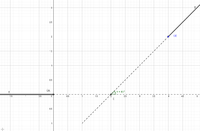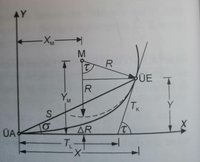# Calculating Clothoid between two tangents

#### Zaragesh

##### New member
I am trying to connect two points with a Clothoid (Euler-Spiral) https://en.wikipedia.org/wiki/Euler_spiral . It is mandatory to connect the points with the correct start and endHeading of the tangents.ÜE and ÜA are member of the tangents g and f
The tangents are intersecting in point E(2,0) and intersecting arc is t = 45° which is also needed for the calculation of the clothoid.
Now some basic Clothoid Formulas I have tried so far: Declaration of FormulaClothoids have the following parameters:
1. the shape parameter A
2. the radius of curvature R at the end point
3. the arc length L
4. the tangent angle t
5. the tangent disengagement deltaR
In order to calculate a clothoid 2 determinants are needed. If a third is added, the clothoid is overdetermined. In my case the tangent angle with t = 45° exists in any case and I mean the shape parameter A must be determined numerically? At the moment I am trying to calculate A iteratively by just increasing it and calculating the Radius with R = A / sqrt(2*t) und L = (A * A)/R. But I never get my Endpoint in that case (4,2) correctly. The Endpoint is calculated by the Solution of the Fresnel Integrals for
X = L * (1-((t * t)/10) * (1-((t * t)/21.6) * (1 - ((t * t)/43.333))));
Y = ((L * t) / 3) * ((1-((t * t)/14) * (1 - ((t * t)/31.429))));
Those formula are correct because I am able to check my solution with a graphic editor software. Does anyone have a solution for this problem or can help me what am I doing wrong?